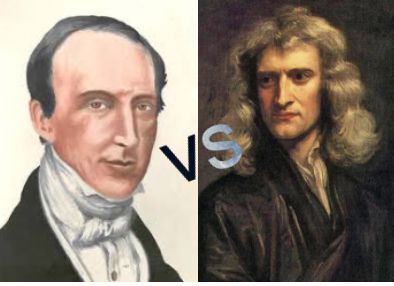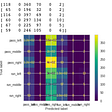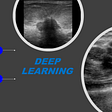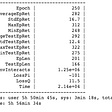# Optimization algorithms in machine learning

## Cauchy vs Newton

The article will focus on optimization algorithms that are interesting to apply in machine learning problems. Newtonian-type algorithms are the most advanced class of optimization algorithms compared to the popular class of gradient descents. But it is the variants of the gradient algorithm that are widely used in machine learning. The fact is that gradient algorithms belong to the so-called Cauchy-type algorithms and have only a linear convergence rate . Augustin Cauchy proposed one of the first variants of the gradient descent algorithm — the steepest descent method (aka the Cauchy method). It uses an iterative procedure in which the alpha k-th step size is estimated using one-dimensional linear optimization, and the s(.) direction is chosen based on the gradient estimate at the k-th point.

More efficient and faster optimizing algorithms with a quadratic convergence rate, such as the Newton method or the Gauss-Newton method, have long been known in theory. However, there are some problems with their application in ML.On the left there`s Augustin Louis Cauchy, on the right — Isaac Newton (portrait by G. Kneller (1689)

For simplicity, let’s consider a variant of the gradient algorithm with a fixed step that demonstrates linear optimization. This algorithm is the basis for any neural network operation. Its advantage lies in the reliability of step-by-step optimization and guaranteed convergence to the suboptimal point. The use of this fairly simple but robust algorithm suggests that we are still at an early stage of applying optimization theory to machine learning.

The fact is that the gradient method applies knowledge about the first derivatives and refers to first-order methods. At the same time, more effective methods for finding optimal solutions have long been developed and exist in the optimization theory. The tasks of training neural networks such as recurrent (RNN), networks with multiple hidden layers (Deep neural networks — DNN), or convolutional networks (CNN) are reduced to finding their weight coefficients. As a rule, one of the variants of the gradient algorithm acts as an optimization algorithm. The options can be seen in the figure.

Thus, it can be argued that all modern machine learning systems are based on a family of gradient algorithms with step-by-step optimization or step-by-step linear solution search. Oddly enough, we are not currently using fast search procedures with a non-linear convergence rate.

An alternative to the gradient algorithm is the Newton method or its options — the modified Newton method . These methods are second-order methods, have quadratic convergence, and use second derivatives. This increases the rate of convergence, achieves a more general search strategy, and accelerates machine learning.

We can compare the convergence rates and the stability of these algorithms on the simplest optimization problem of a function that depends on two parameters [x,y].

Let`s consider an example of a multimodal function with several local minima, given by the following expression:

This is the Himelblau`s function that`s well known in optimization theory. It has four adjacent local minima, one central local maximum, and four saddles.

The function is interesting from the point of view of finding optimal points and testing training or optimization algorithms. The code for plotting the Himmelblau`s function looks as follows:

The problem of finding optimal coefficients (optimal solution of the objective function) for a neural network is that modern problems have large dimensions of N > 1000. The situation is complicated by heterogeneous, “raw” training data, which leads to noise on the target function surface. For example, adding normal-distribution noise with parameters M=0, sigma =20 to the Himmelblau`s function leads to a significant distortion of the surface. This is the reason for the malfunction of the numerical algorithm and the appearance of errors in the estimation of the neural network coefficients.

Practice shows that modern versions of the gradient algorithm with adaptive step adjustment, constraints, penalty functions, and regularization allow to adjust the network weight coefficients quite accurately.

Let’s understand why the simpler version of the gradient method does not work effectively and requires so many complications. We plot the convergence graphs of the gradient algorithm and the Newton method to the optimal point for the reduced Himmelblau`s function.

The code of the gradient algorithm with search logging is presented below.

W_history variables are the values of the function’s arguments, f_history are values of the function, eps_history_f is the norm distance between the values of the functions, eps_history_xy is the norm distance between the values of the argument. These sets of variables are stored in stacks using np. stack(). They allow to analyze the convergence of the gradient algorithm to the optimal solution f (x*,y*) for the Himmelblau`s function f(x,y). The convergence to the optimal solution can be expressed by the limit.

Similarly in machine learning, the gradient algorithm minimizes the target error function J(.) from the vector of theta parameters in the same way. It fits the h hypothesis to the data training data.

Let’s present the convergence graphs obtained for the f (x,y) function and the [x,y] arguments.

The graphs demonstrate the convergence of the gradient algorithm in ~200 iterations. For comparison, Newton’s method converges in just ~20 iterations. The Newton’s method is presented below.

The gradient method slowly slides to one of the optimal points with coordinates [3, 2]. The Newton method, on the contrary, jumps along the surface of the target function in the direction of the same optimum.

Why not use second-order methods in machine learning? After all, they allow to apply a more general search strategy. The fact is that despite their effectiveness, second-order methods are less stable. They give a number of outliers in the adaptation process. When using noisy target functions in real-world situations, neural networks will be trained with even more outliers.

The optimization of the noisy Himmelblau`s function, where both the function value and the value of the first gradient are noisy by Gaussian white noise with parameters (M=0, sigma =5), can be observed below.

Graphs of convergence to the suboptimal point for the noisy function show that the slow gradient algorithm looks more preferable than the fast “Newton”!

The Newton method has time to adjust to the noise jitter of the function, which negatively affects the estimation of the optimal values of [x,y] (green graphs in the figure below). At the same time, the gradient method gives a fairly accurate average estimate of the arguments (red graphs for[x,y] below).

Thus, it is quite difficult to identify a more reliable and faster algorithm when optimizing the cost function J for a neural network. Engineers usually choose a slow but reliable step-by-step gradient algorithm with a number of modifications called Adam  or its second version called Nadam . Since the real data are very noisy and not homogeneous, the slow convergence property of the gradient algorithm is very useful. The full code with comments and many parameters for comparing the Cauchy algorithm (the basic gradient descent algorithm) and Newton can be found at:

1. Rekleytis G., Reyvindran A., Regsdel K. (1986). Optimization in the Equipment. In 2 books. Book 1. Translation from English. M.: Mir (in Russian).

--

--

--

## More from Analytics Vidhya

Analytics Vidhya is a community of Analytics and Data Science professionals. We are building the next-gen data science ecosystem https://www.analyticsvidhya.com

## Final_McComas_Drew## Deep learning for improved breast cancer monitoring using a portable ultrasound scanner## How to build a deep learning GPU workstation while saving \$1500?## In this blog post I will be discussing about K-Nearest Neighbour.K-nearest## Grouped Convolutions — convolutions in parallel## Steps to building a Poker AI — Part 2: Modelling Imperfect Information Games## Enable GPU for Soft Actor Critic with 4 lines of codes## Alexey Titov

R&D engineer in machine learning and data analysis. Java, C/C++, Python, M and CUDA. HPC, processors architectures and parallel systems.

## Interview With AI Researcher: How to become one and what do they do## All about Data Splitting, Feature Scaling and Feature Encoding in Machine Learning